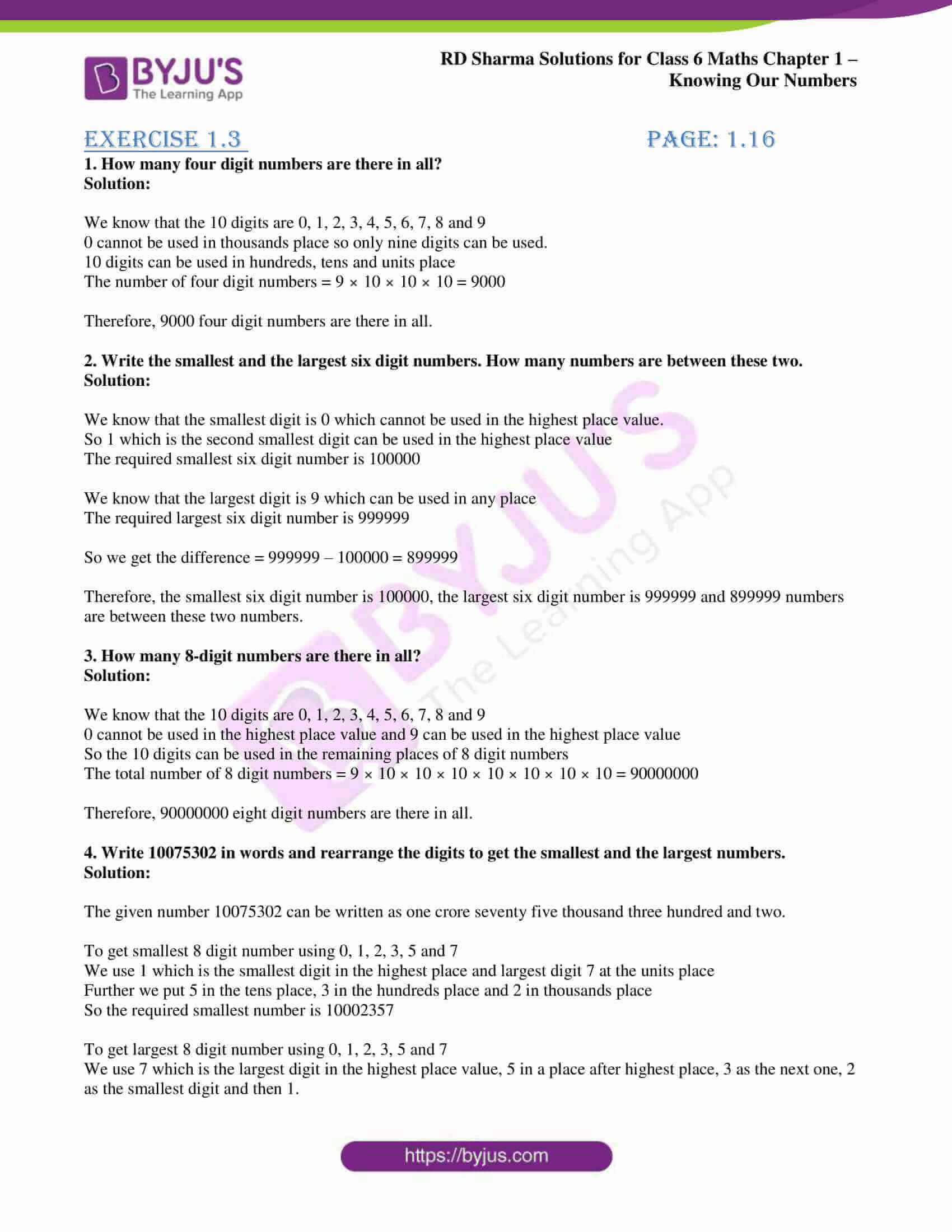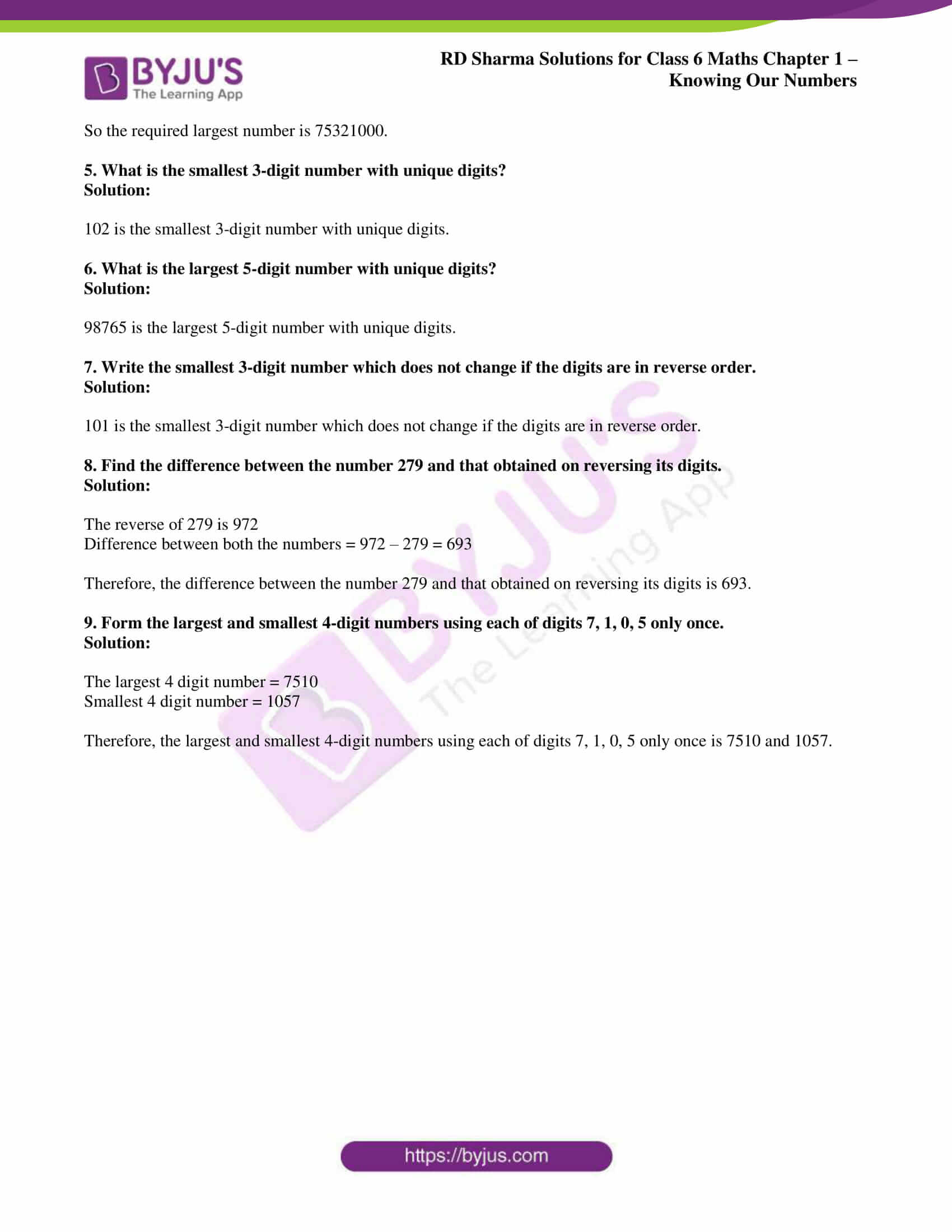# RD Sharma Solutions for Class 6 Maths Chapter 1: Knowing Our Numbers Exercise 1.3

## RD Sharma Solutions for Class 6 Maths Exercise 1.3 PDF

The primary aim of creating solutions for all the exercises is to make the subject easy for students. This helps in improving the self study method with the help of interactive explanations given in each solution. The students while solving the problems of RD Sharma textbook can use PDF of solutions as a reference guide. The solutions contain various methods of solving each problem, which help students to obtain good marks. The PDF of solutions can be downloaded effortlessly by students in order to improve logical thinking abilities.
RD Sharma Solutions for Class 6 Maths Chapter 1 Knowing Our Numbers Exercise 1.3 are provided here.

## RD Sharma Solutions for Class 6 Maths Chapter 1: Knowing Our Numbers Exercise 1.3 Download PDF## Access answers to Maths RD Sharma Solutions for Class 6 Chapter 1: Knowing Our Numbers Exercise 1.3

1. How many four digit numbers are there in all?

Solution:

We know that the 10 digits are 0, 1, 2, 3, 4, 5, 6, 7, 8 and 9

0 cannot be used in thousands place so only nine digits can be used.

10 digits can be used in hundreds, tens and units place

The number of four digit numbers = 9 × 10 × 10 × 10 = 9000

Therefore, 9000 four digit numbers are there in all.

2. Write the smallest and the largest six digit numbers. How many numbers are between these two.

Solution:

We know that the smallest digit is 0 which cannot be used in the highest place value.

So 1 which is the second smallest digit can be used in the highest place value

The required smallest six digit number is 100000

We know that the largest digit is 9 which can be used in any place

The required largest six digit number is 999999

So we get the difference = 999999 – 100000 = 899999

Therefore, the smallest six digit number is 100000, the largest six digit number is 999999 and 899999 numbers are between these two numbers.

3. How many 8-digit numbers are there in all?

Solution:

We know that the 10 digits are 0, 1, 2, 3, 4, 5, 6, 7, 8 and 9

0 cannot be used in the highest place value and 9 can be used in the highest place value

So the 10 digits can be used in the remaining places of 8 digit numbers

The total number of 8 digit numbers = 9 × 10 × 10 × 10 × 10 × 10 × 10 × 10 = 90000000

Therefore, 90000000 eight digit numbers are there in all.

4. Write 10075302 in words and rearrange the digits to get the smallest and the largest numbers.

Solution:

The given number 10075302 can be written as one crore seventy five thousand three hundred and two.

To get smallest 8 digit number using 0, 1, 2, 3, 5 and 7

We use 1 which is the smallest digit in the highest place and largest digit 7 at the units place

Further we put 5 in the tens place, 3 in the hundreds place and 2 in thousands place

So the required smallest number is 10002357

To get largest 8 digit number using 0, 1, 2, 3, 5 and 7

We use 7 which is the largest digit in the highest place value, 5 in a place after highest place, 3 as the next one, 2 as the smallest digit and then 1.

So the required largest number is 75321000.

5. What is the smallest 3-digit number with unique digits?

Solution:

102 is the smallest 3-digit number with unique digits.

6. What is the largest 5-digit number with unique digits?

Solution:

98765 is the largest 5-digit number with unique digits.

7. Write the smallest 3-digit number which does not change if the digits are in reverse order.

Solution:

101 is the smallest 3-digit number which does not change if the digits are in reverse order.

8. Find the difference between the number 279 and that obtained on reversing its digits.

Solution:

The reverse of 279 is 972

Difference between both the numbers = 972 – 279 = 693

Therefore, the difference between the number 279 and that obtained on reversing its digits is 693.

9. Form the largest and smallest 4-digit numbers using each of digits 7, 1, 0, 5 only once.

Solution:

The largest 4 digit number = 7510

Smallest 4 digit number = 1057

Therefore, the largest and smallest 4-digit numbers using each of digits 7, 1, 0, 5 only once is 7510 and 1057.

### RD Sharma Solutions Class 6 Maths Chapter 1 – Knowing Our Numbers Exercise 1.3

RD Sharma Solutions Class 6 Maths Chapter 1 Knowing Our Numbers Exercise 1.3 has answers to the exercise based on finding the smallest and largest numbers in the given set of information.

### Key features of RD Sharma Solutions for Class 6 Maths Chapter 1: Knowing Our Numbers Exercise 1.3

• Exam preparation can be done by making use of exercise wise solutions created by faculty at BYJU’S.
• The students gain more knowledge of concepts which is important in solving exercise wise problems.
• The problems of RD Sharma textbook are based on frequently asked questions in previous exams.
• The students can use PDF for revision purpose to understand their areas of weaknesses.